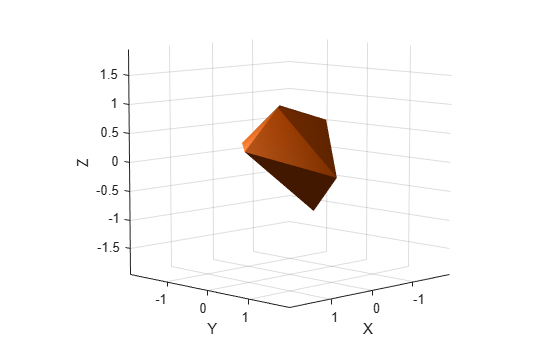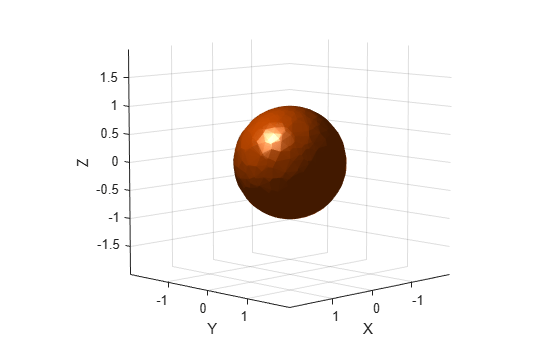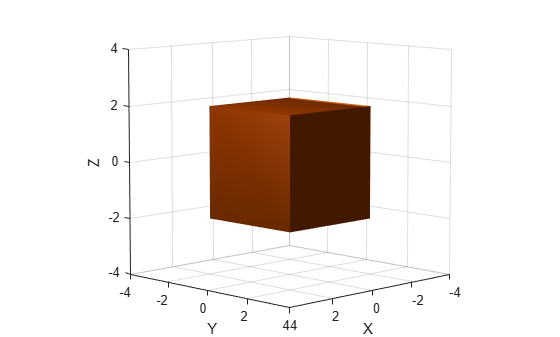# collisionMesh

Create convex mesh collision geometry

Since R2019b

## Description

Use `collisionMesh` to create a collision geometry as a convex mesh.

## Creation

### Syntax

``MSH = collisionMesh(Vertices)``
``MSH = collisionMesh(___,Pose=pose)``

### Description

example

````MSH = collisionMesh(Vertices)` creates a convex mesh collision geometry from the list of 3-D Vertices. The vertices are specified relative to a frame of choice (collision geometry frame). By default, the collision geometry frame collocates with the world frame.```
````MSH = collisionMesh(___,Pose=pose)` sets the `Pose` property of the mesh to `pose`, relative to the world frame.```

## Properties

expand all

Vertices of a mesh, specified as an N-by-3 array, where N is the number of vertices. Each row of `Vertices` represents the coordinates of a point in 3-D space. Note that some of the points can be inside the constructed convex mesh.

Data Types: `double`

Pose of the collision geometry relative to the world frame, specified as a 4-by-4 homogeneous matrix or an `se3` object. You can change the pose after you create the collision geometry.

Note

Note that when the pose is specified as an `se3` object, the `Pose` property stores the pose as a numeric 4-by-4 matrix.

Data Types: `single` | `double`

## Object Functions

 `show` Show collision geometry `fitCollisionCapsule` Fit collision capsule around collision geometry

## Examples

collapse all

Create an array consisting of the coordinates of ten points randomly chosen on the unit sphere. For reproducibility, set the random seed to the default value.

```rng default n = 10; pts = zeros(n,3); for k = 1:n ph = 2*pi*rand(1); th = pi*rand(1); pts(k,:) = [cos(th)*sin(ph) sin(th)*sin(ph) cos(ph)]; end```

Create a convex mesh collision geometry from the array. Visualize the collision geometry.

```m = collisionMesh(pts); show(m)```Create a second array similar to the first, but this time consisting of 1000 points randomly chosen on the unit sphere.

```n = 1000; pts2 = zeros(n,3); for k = 1:n ph = 2*pi*rand(1); th = pi*rand(1); pts2(k,:) = [cos(th)*sin(ph) sin(th)*sin(ph) cos(ph)]; end```

Create and visualize a mesh collision geometry from the array. Observe that choosing more points on the sphere results in a sphere-like mesh.

```m2 = collisionMesh(pts2); show(m2)```Create an array consisting of the coordinates of the eight corners of a cube. The cube is centered at the origin and has side length 4.

```cubeCorners = [-2 -2 -2 ; -2 2 -2 ; 2 -2 -2 ; 2 2 -2 ;... -2 -2 2 ; -2 2 2 ; 2 -2 2 ; 2 2 2]```
```cubeCorners = 8×3 -2 -2 -2 -2 2 -2 2 -2 -2 2 2 -2 -2 -2 2 -2 2 2 2 -2 2 2 2 2 ```

Append `cubeCorners` to `pts2`. Create and visualize the mesh collision geometry from the new array. Because the cube contains the sphere, the sphere points that are interior to the cube are disregarded when creating the geometry.

```pts3 = [pts2;cubeCorners]; m3 = collisionMesh(pts3); show(m3)```## Version History

Introduced in R2019b

expand all